# Modeling porous electrodes: Part 2

A secondary current distribution model considers:

1. Ohm’s law resistance, and
2. kinetic charge-transfer resistance

while resistance related to concentration variations is ignored. Beginning with the equations given in Modeling porous electrodes: Part 1, the following can be written: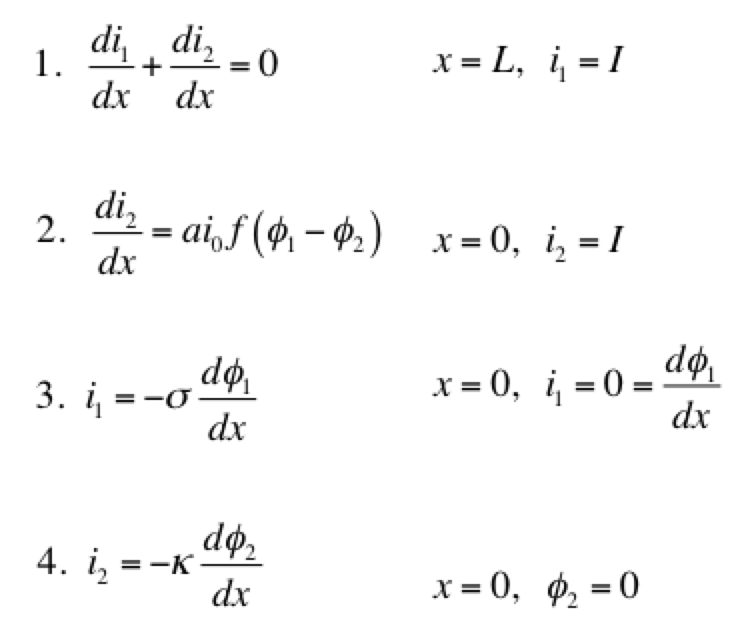Eq 1 is conservation of charge; Eq 2 is some expression of electrochemical reaction kinetics; and Eqs 3 & 4 are Ohm’s law for the solid and liquid phases. The boundary conditions are such that a current density of I is being produced by the electrode. The liquid phase potential φ2 is arbitrarily set to 0 V at x = 0. The specific expression for the electrode kinetics (Eq 2) needs to be specified. The concentration-independent Butler-Volmer expression can be used: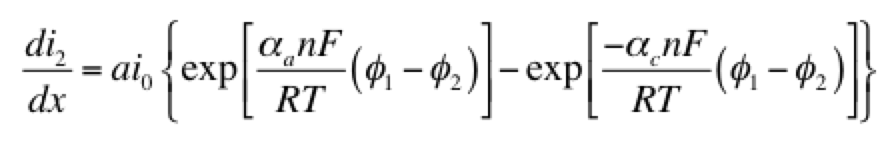The left hand side of this expression is the transfer current, with units of A/cm3, and gives the amount of electrochemical reaction occurring at any point. The right hand side has two components: the anodic and cathodic kinetic terms. The downside of the Butler-Volmer equation is its complexity. Finding an analytical solution to the above set of differential equations requires a more simplified kinetic expression. One possible simplification is to assume the overpotential (φ– φ2) is highly negative. This means only the cathodic term in the Butler-Volmer equation need be considered: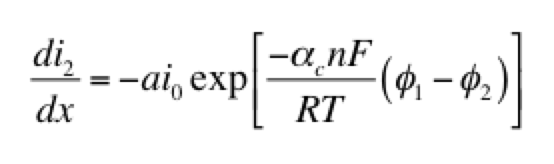The right hand side of this expression is a form of the Tafel equation. (An analogous anodic form can be used when overpotential is highly positive.) Another possible simplification is to assume the overpotential (φ1 – φ2) is small. In this case both anodic and cathodic terms are important. Substituting the first two terms of a Taylor series for the exponentials results in: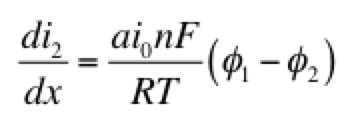This linear kinetic expression is valid only at low currents. Which approximation of the kinetic expression is appropriate depends on the conditions of interest. Choosing the following values for physical parameters:

• electrode area per volume, a = 23,300 cm-1
• transfer coefficients, αaαc = 0.5
• exchange current density, i0 = 2 x 10-7 A/cm2
• current density, I = 0.1 A/cm2
• length, L = 1 cm
• electrolyte conductivity, κ = 0.06 S/cm
• electrode conductivity, σ = 20 S/cm

we assume that the Tafel approximation for kinetics will be valid, because the current density is much larger than the exchange current density. Solving the set of equations 1-4 for both Tafel and linear kinetics gives the results below: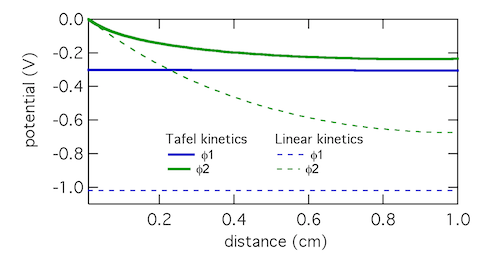The Tafel kinetic expression predicts that current exchange from liquid to solid phases (ionic current to electronic current) will be concentrated near x = 0, the location closest to the counter electrode. There (φ1 – φ2) will be near 300 mV, falling away further into the electrode. In contrast, the linear kinetic expression predicts a more even exchange of current through the electrode, and much higher values of (φ1 – φ2). This is because the linear expression is a softer function of overpotential than the exponential one. The Tafel approximation is clearly the more appropriate in this case because (φ1 – φ2) » RT/F which is 25.7 mV at room temperature.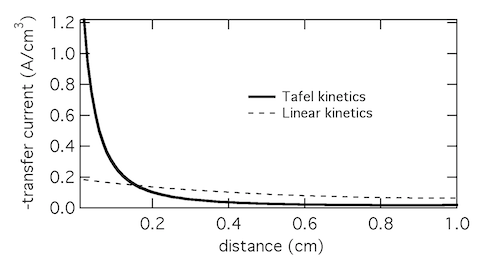Plotting the transfer current di2/dx shows that in reality the electrochemical reaction is concentrated in the front 10% of the electrode. Linear kinetics tend to result in more uniform current distributions, but for this to be the case i0 > I will need to hold.

Click here for Modeling porous electrodes: Part 3, which is about solving porous electrode problems using Newman’s BAND method.

# High energy battery characterization

We have a new paper out, which describes work using high energy battery characterization to map the insides of batteries without opening them. “Hetaerolite Profiles in Alkaline Batteries Measured by High Energy EDXRD” is in the current issue of the Journal of the Electrochemical Society. The idea is to use X-rays with high energy and high flux to penetrate a thick battery and collect diffraction data. By setting slits to collimate the X-ray beam, a well-defined gauge volume (GV) is examined within the battery, as illustrated below: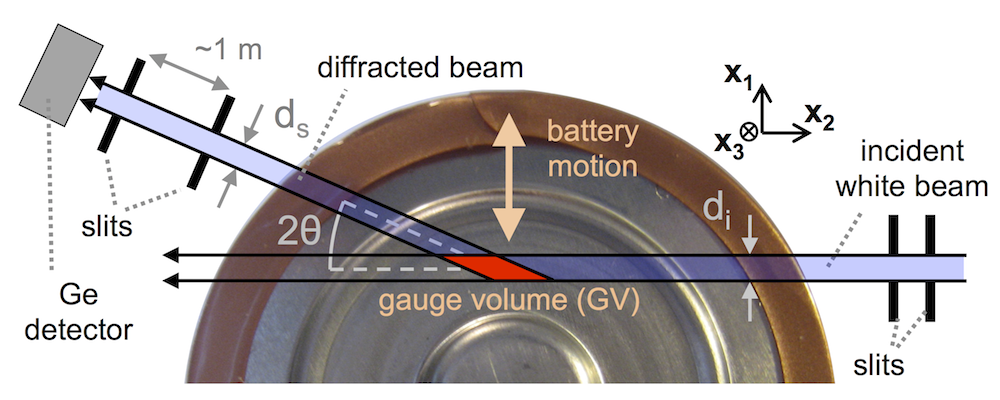Technically this is called energy dispersive X-ray diffraction or EDXRD. For each sample of data, you obtain three pieces of information: 1) Energy of the photons diffracted, 2) Intensity of the photons diffracted, and 3) Physical location inside the battery. This is plotted as a “diffraction contour” with energy on the x-axis, physical location on the y-axis, and log intensity of the diffraction as pixel darkness. As different crystalline materials diffract X-rays of different energy, you get an XRD fingerprint of the materials. Techniques like this are revolutionizing battery analysis, because physically opening a battery results in unacceptable mixing and oxidation.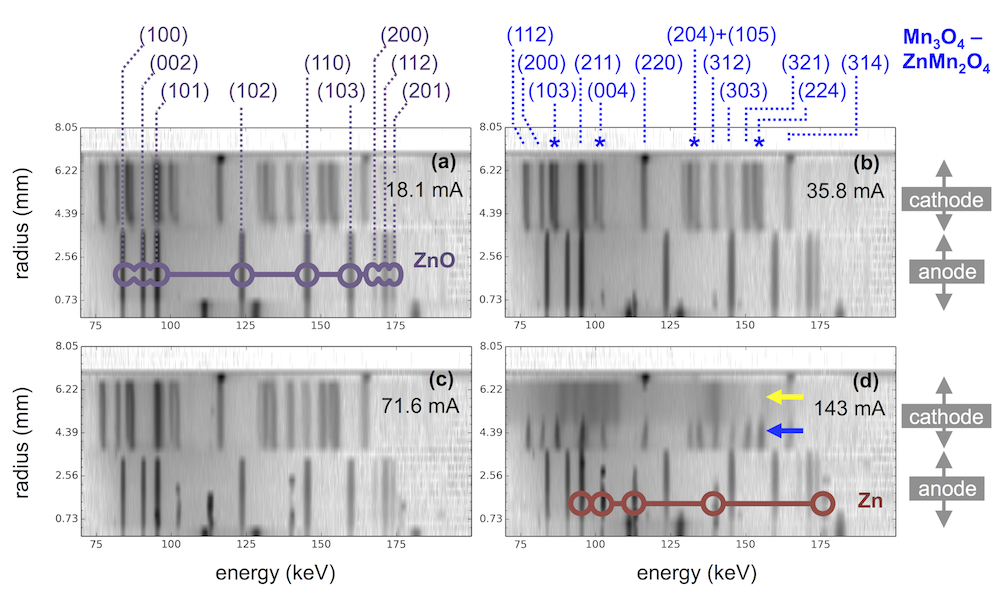The plot above shows diffraction contours for four AA sized alkaline batteries discharged at different rates. The anodes are mixtures of zinc and zinc oxide, with higher current leaving more zinc behind. The cathodes are mostly mixtures of Mn3O4 and ZnMn2O4, which are nearly identical materials called hausmannite and hetaerolite. Even though these materials have structures differing by only 0.04 angstroms, EDXRD distinguishes them via peak splitting, marked above by blue stars.

The interesting thing is that the highest discharge rate (143 mA) has no hausmannite, but is instead split between hetaerolite and a different material, MnOOH. This shows that these two materials are the first to form, and that hausmannite is only a later product. As discussed in another recent paper, we want to know what reaction pathway leads to hetaerolite, because it limits the rechargeable performance of alkaline batteries. The results above provide the best clue we’re aware of, suggesting there is a groutite -> hetaerolite transition.

# Extremely inexpensive grid-scale batteries

We have a new paper out, dealing with our long-term effort to develop extremely inexpensive and safe batteries for large-scale use. The full title is “Rechargeability and economic aspects of alkaline zinc-manganese dioxide cells for electrical storage and load leveling.” The idea driving the research is that since DOE cost targets for grid-scale storage are very low (\$100/kWh), battery design should begin with the cheapest and safest materials possible, instead of trying to adapt high-power lithium-ion battery designs.

Zinc and manganese dioxide fit that bill, and what the paper shows is that by reducing the depth of discharge (DOD) to some fraction of the full capacity, long cycle life can be achieved at extremely low cost. The plot below shows that at 10% DOD over 3000 cycles are typical, while at 20% DOD it is over 500. These are base designs, focusing on electrode construction, materials, porosity, etc, with no additives or specialty materials. Assuming one cycle a day, this is an 8.5 year lifespan at 10% DOD.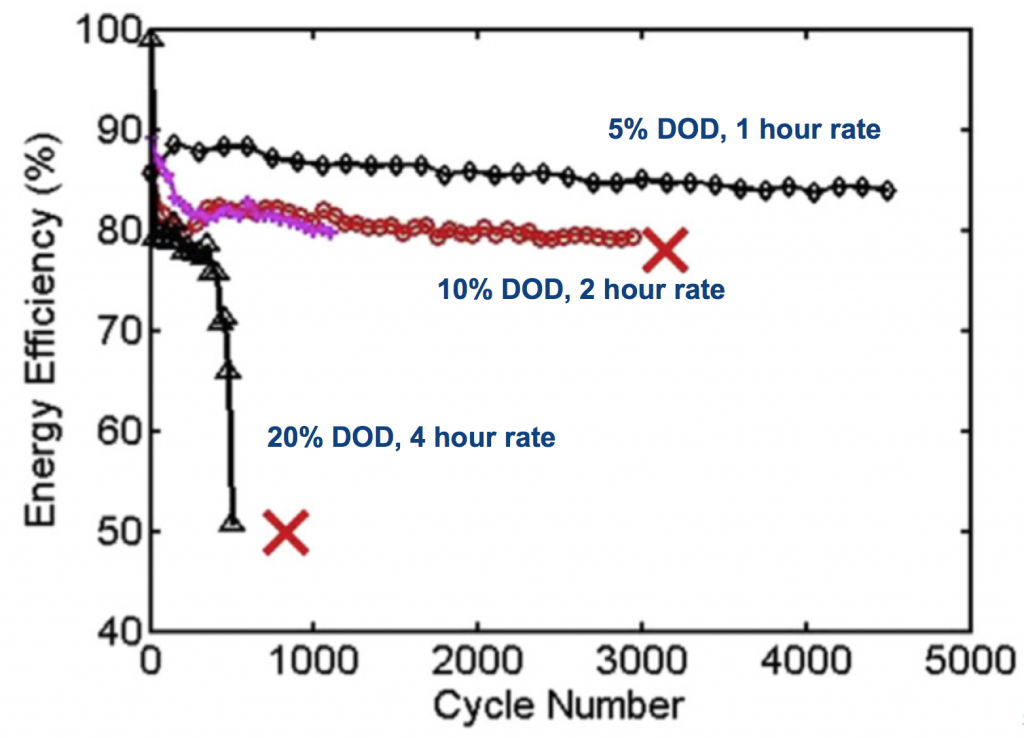Cycling a fraction of the DOD like this allows a long lifespan, while keeping the cost low. This shallow-cycled Zn-MnO2 battery comes in very low in lifetime investment cost, 2-5 ¢/kWh/cycle. Using numbers taken from the literature, this is in or below the projected range of vanadium-redox and sodium-metal-halide (ZEBRA) batteries, while being low-temperature and generally lower complexity than these. An extra benefit is that since only a fraction of the Zn-MnO2 capacity is cycled, the reserve capacity can be used in emergencies.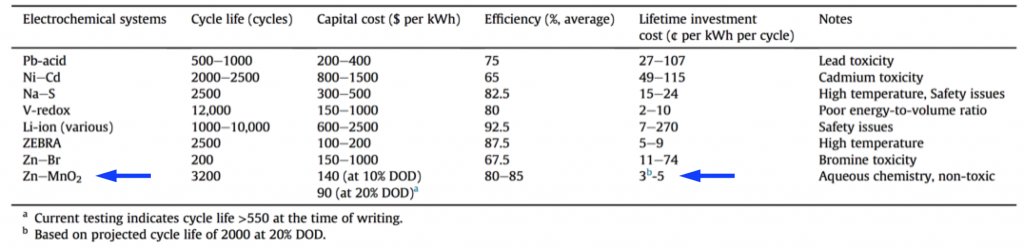Over the last few years we’ve developed these types of batteries in many shapes and sizes, supported by DOE’s ARPA-E program as well as other entities like the utility Con Edison. A couple of battery racks in the CUNY lab are shown below. A little over a year ago Urban Electric Power LLC was spun out, and in their battery labs a few blocks away on 127th Street they’ve been developing the batteries for commercialization, and refining the design for higher power and longer life.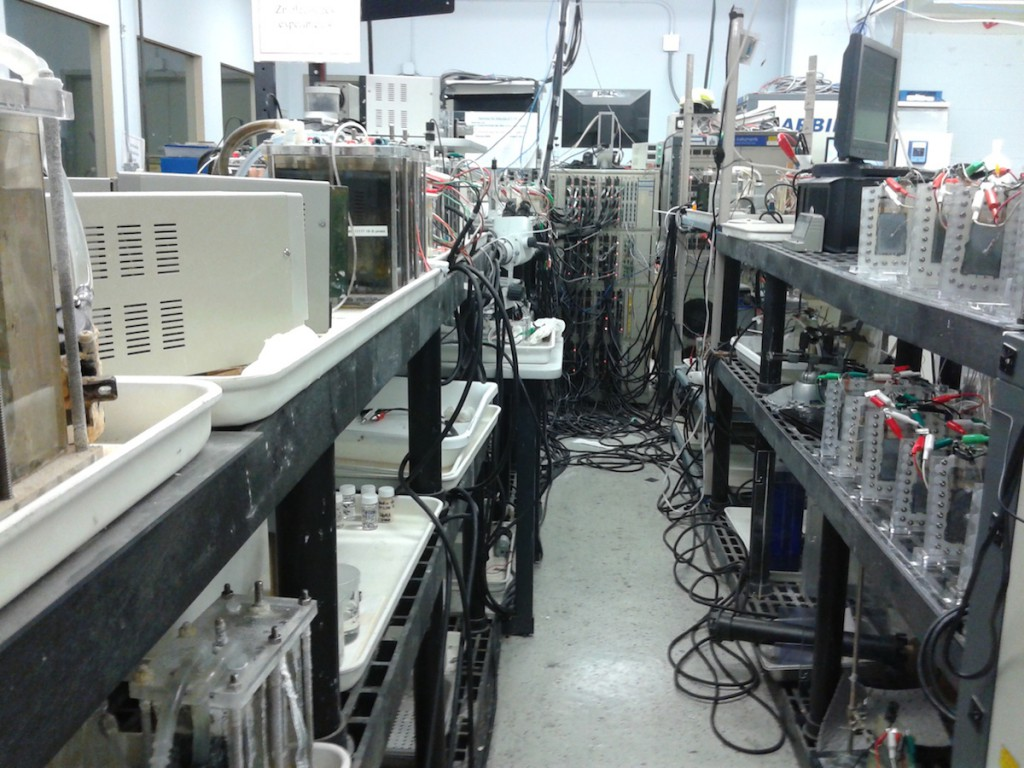Our analysis in the paper addresses evolution of the batteries during their lifetime. If you notice above, at 10% DOD the efficiency falls from near 90% to near 80% during cycling, due to a kind of “formation” of the materials. The analysis, which was Nilesh Ingale’s doctoral thesis, is too dense to describe in full but a combined modeling+experimental effort shows that the MnO2 active particles in the cathode become coated with hetaerolite (ZnMn2O4) and this resistive film drops performance. Below you see this resistivity plotted against cycle number, showing the effect mostly occurs in the first 150 cycles. Preventing this will help efficiency, but could also impact how the batteries are made and lower cost.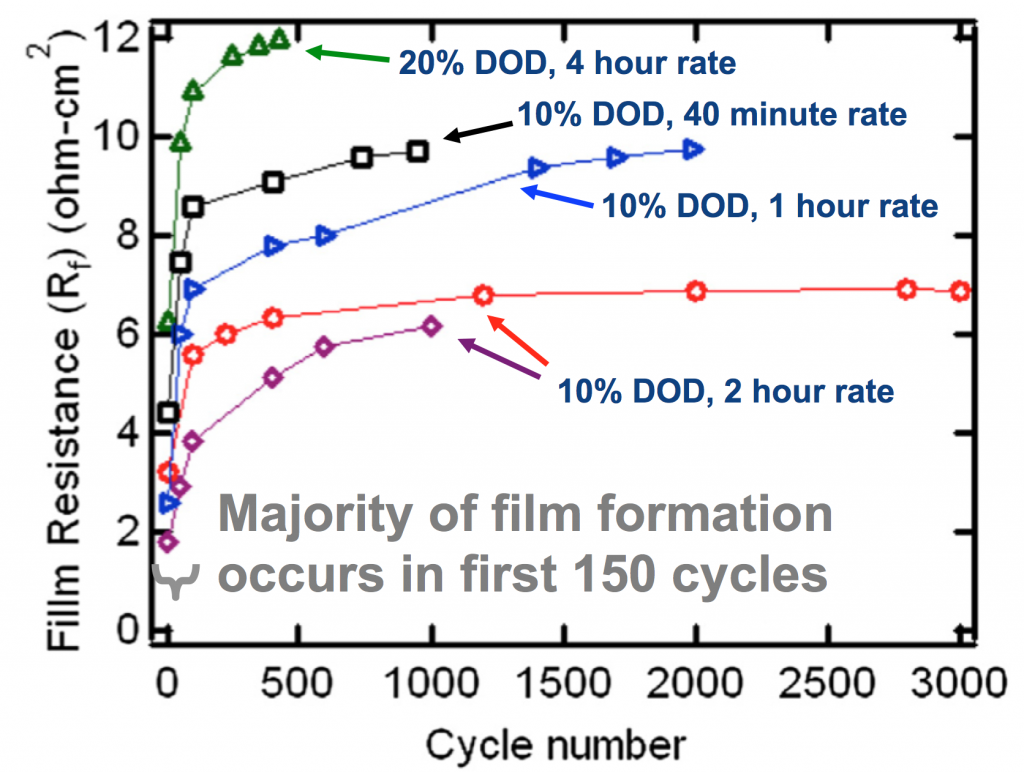# Modeling porous electrodes: Part 1

Useful electrochemical devices often employ porous electrodes. This is because electrochemistry is by definition an interfacial phenomenon, usually happening where a liquid and a solid meet. Porous electrodes offer a high surface area, allowing a lot of reaction to be packed into a small space.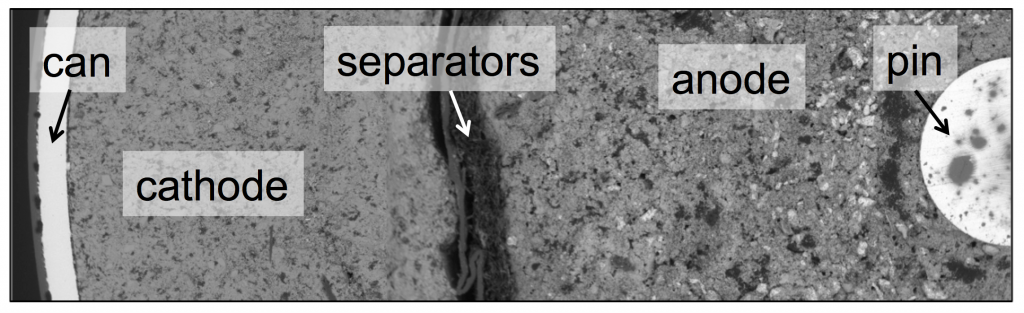The SEM image above shows the cross-section of a Duracell AA battery, contained by a cylindrical can, with a pin at the center. The two electrodes, cathode and anode, take up most of the space inside. Both electrodes are made of particles, with liquid electrolyte filling the pores in between. A porous separator keeps the cathode and anode from touching each other and shorting.

Porous electrode theory was developed in the 1950s and 1960s to allow modeling of these kinds of electrochemical devices, and it has been successful at explaining many of the empirical facts one observes when using them. Much cutting-edge electrochemical engineering continues to rely on porous electrodes, from bioelectrochemical cells to the batteries in the Tesla Model S. Porous electrode theory is well understood, but even the most modern textbook treatments resemble journal articles in how they are structured and written. This post is an attempt to describe the theory in an accessible way. Fundamentally, the problem is a highly relevant form of transport phenomena, and should be understandable to a wider engineering audience.

A simple porous electrode model:

Ultimately a battery like the one above is part of an electrical circuit, and current must travel across the cell, from pin to can. We call the pin and can “current collectors” because they don’t participate in reactions, and are there to connect the circuit to the anode and cathode. If porous electrodes are designed well, there are ample conduction pathways through both the particle (solid) and electrolyte (liquid) phases. “Current” is the movement of charge. In solids, charge typically moves as electrons, which are negatively charged. In liquids, charge moves as ions, which may be positive or negative.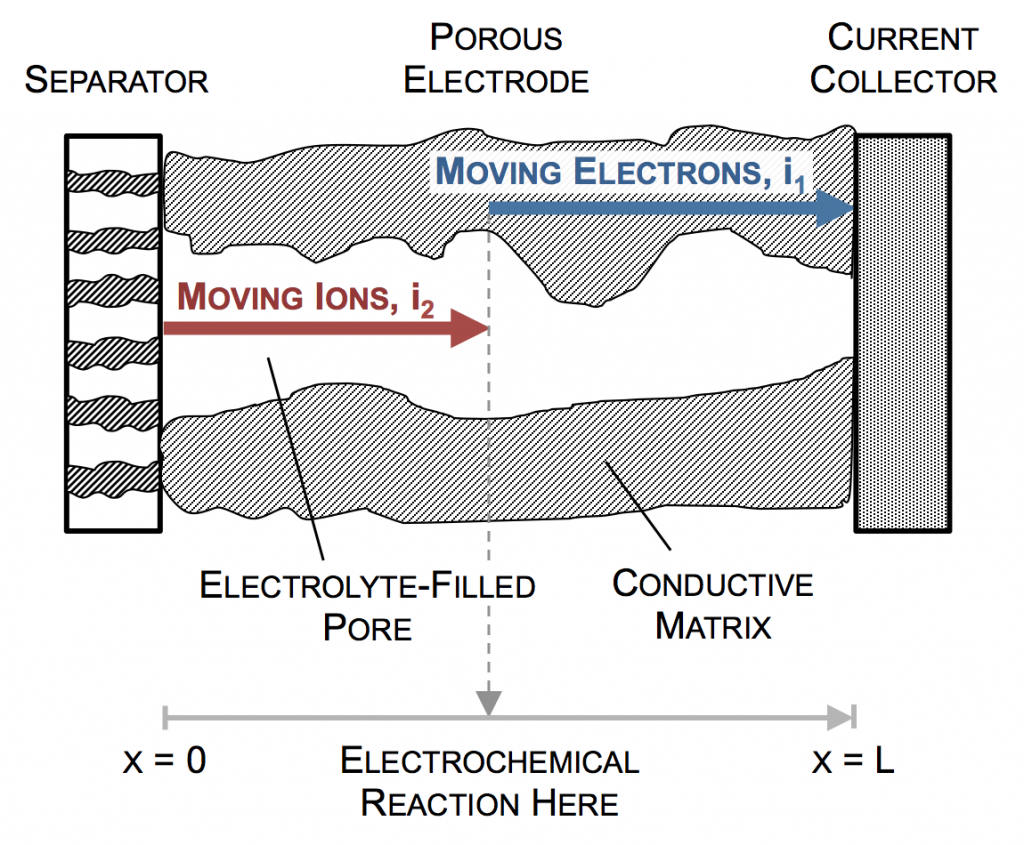In the picture above, the complex network of pores through an electrode are described as a single pore, which extends from separator to current collector. The direction across the electrode is given by x, and we use rectangular coordinates to keep the math simple. (Thus, this is one electrode or half of the battery above, but in a rectangular configuration instead of a cylindrical one.)

The separator will not allow electrons to pass, and thus only ionic current can come through at x = 0. The current collector will not allow ions to pass, so only electrons can flow out at x = L. This is the essence of the porous electrode model: the total current flow from separator to current collector is constant: the current across the circuit, denoted as I. However, across the porous electrode it may travel either as ions or as electrons. The only necessity is that it must be all-ionic at the separator and all-electronic at the current collector.

Ionic current and electronic current may be converted to one another by an electrochemical reaction. In fact, this is the definition of electrochemistry: the study of reactions that involve both chemicals (ions) and electricity (electrons). In the picture above a unit of ionic current ends at the same location where one of electronic current begins. In reality, the ion causing the ionic current turns upward, contacts the pore wall, and there participates in the liberation of an electron. But for simplicity, we draw the entire thing as involving only motion in the x-direction.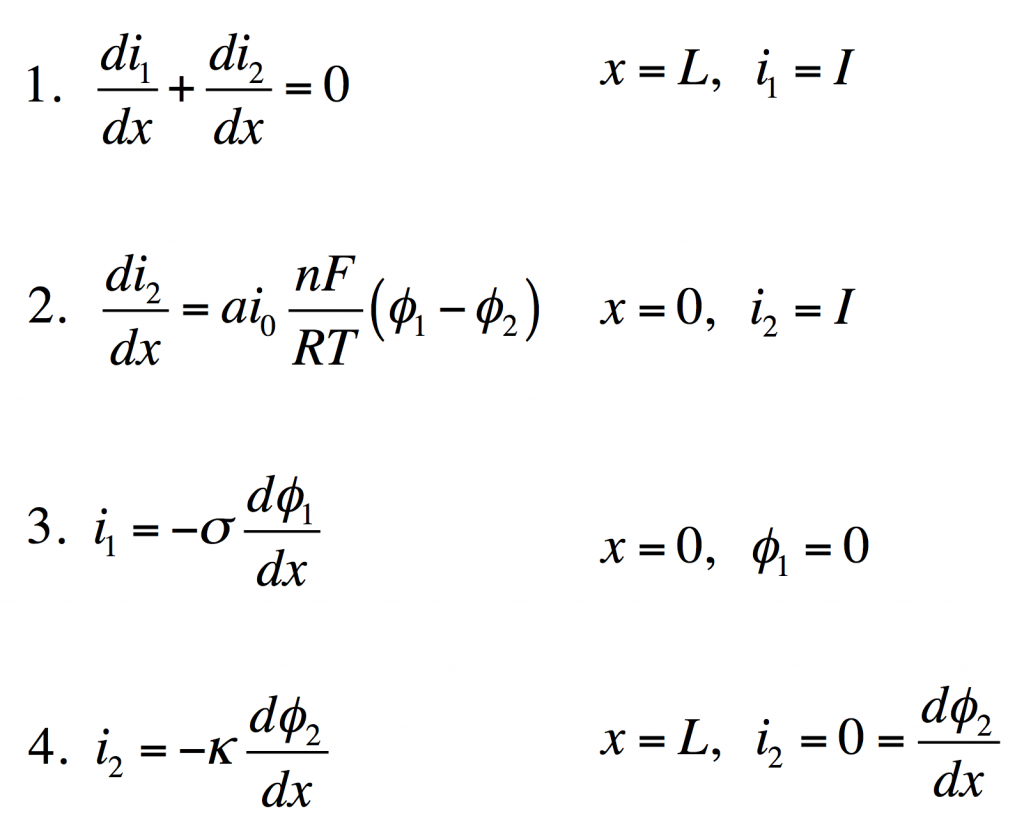These four equations describe a steady-state porous electrode operating this way, with boundary conditions given on the right. The four unknowns are i1 and i2, the electronic and ionic currents at any x, and φ1 and φ2, the electric potentials in the solid and the liquid.

1. Conservation of charge. The equation states that any i2 that disappears must be matched by appearance of i1 and vice versa. The boundary condition states all current is electronic at the current collector.
2. Rate of electrochemical reaction. The equation states that i2 disappears by an interfacial reaction that is driven (linearly) by the potential difference between the solid and liquid. The boundary condition states all current is ionic at the separator.
3. Ohm’s law in the solid. This relates current to potential gradient. The boundary condition arbitrarily sets the solid potential to zero at the separator.
4. Ohm’s law in the liquid. The boundary condition states the liquid potential gradient is zero at the current collector.

These can be manipulated into a single equation: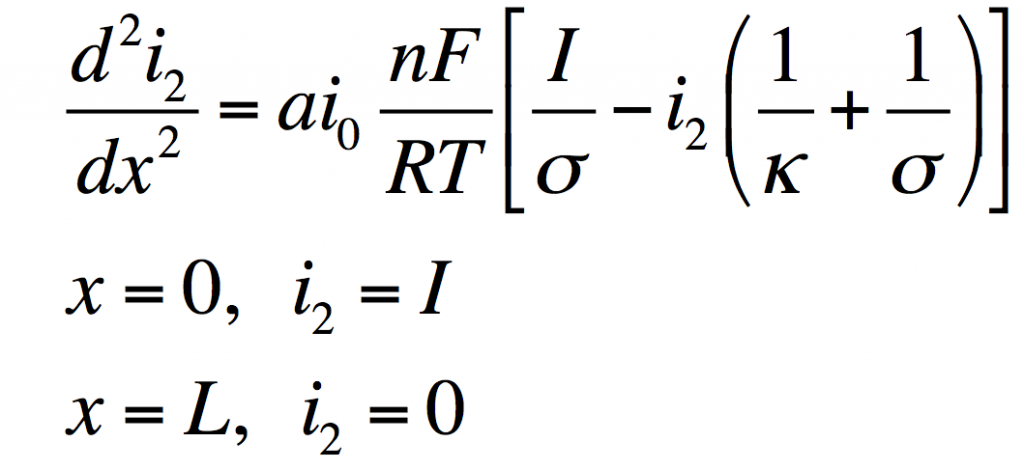This has the solution: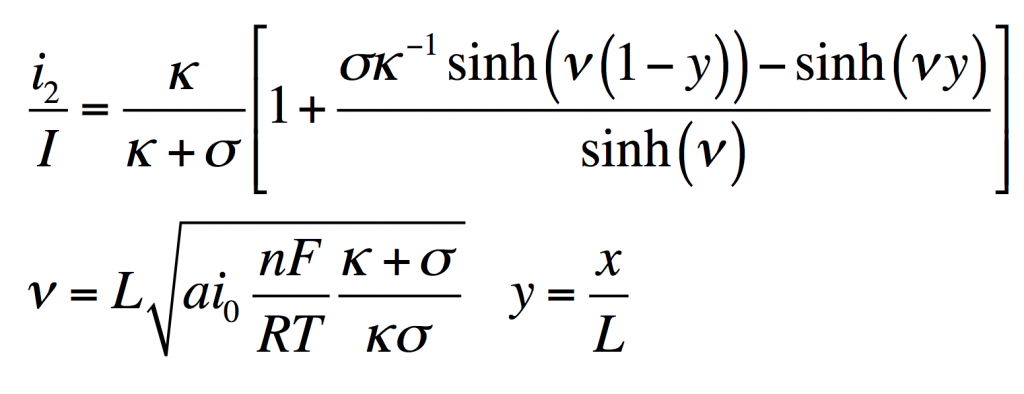From this i1φ1, and φ2 can be found by substitution into the original equations.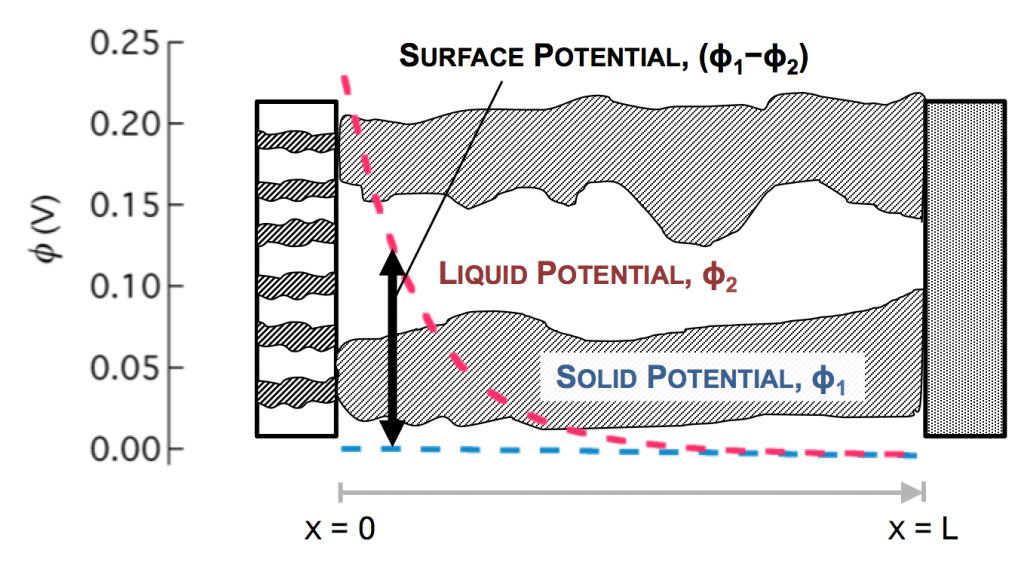Values typical for a porous battery electrode can be used to plot the variables:

• electrode area per volume, a = 23,300 cm-1
• exchange current density, i0 = 3.5 x 10-6 A/cm2
• current density, I = 0.1 A/cm2
• length, L = 1 cm
• electrolyte conductivity, κ = 0.06 S/cm
• electrode conductivity, σ = 20 S/cm

The disparity in conductivities results in the general behavior we observe. The solid potential φ1 changes very little due to its high conductivity. However, in the liquid there is a large change in φ2 near the separator. The reaction driving force in equation 2 is the difference in these potentials, the surface potential. Thus there is substantially more reaction near the separator than elsewhere in the electrode.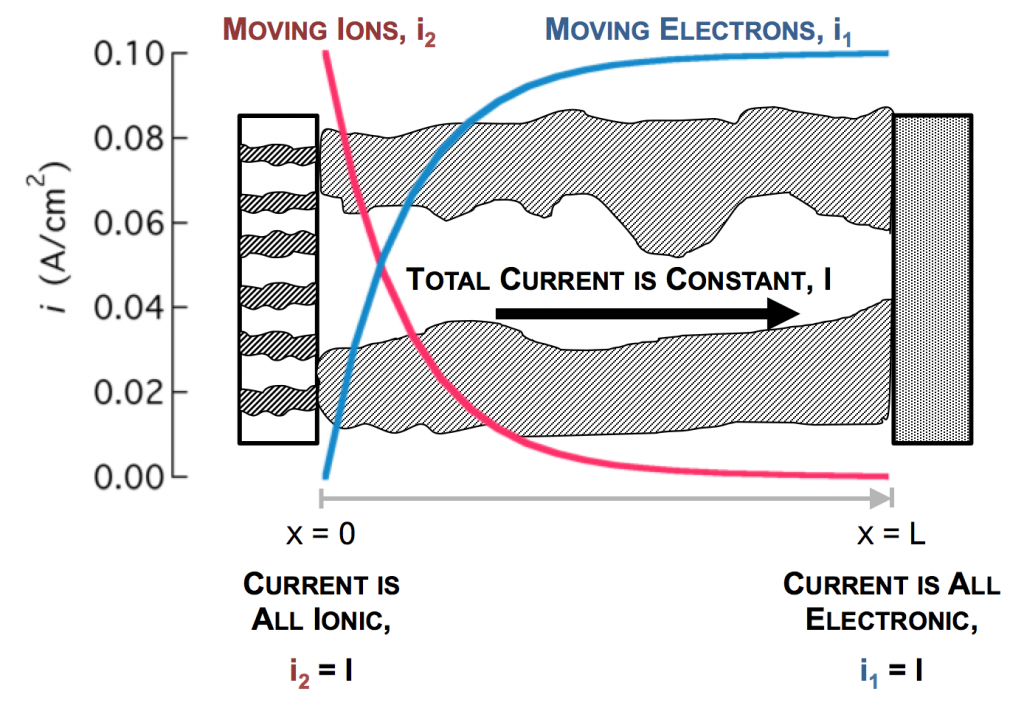As the reaction causes conversion of i2 to i1 the current profiles change greatly near the separator. The total current is always i1 + i2 = I at any point.

Click here for Modeling porous electrodes: Part 2, which is about the difference between linear and Tafel kinetics.

Click here for Modeling porous electrodes: Part 3, which is about solving porous electrode problems using Newman’s BAND method.